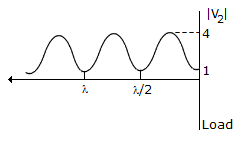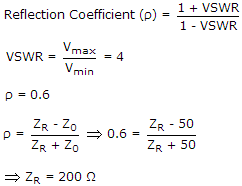# Electronics and Communication Engineering - Electromagnetic Field Theory

46.

Cassergain feed is used with a parabolic reflector to

 A. increase the gain of the system B. increase the beamwidth of the system C. reduce the size of the main reflector D. allow the feed to be placed at a convenient point

Explanation:

Cassergain is a type of feed, which is used to allow parabolic reflector to provide feed at a convenient point.

47.

Voltage standing wave pattern in a loss less transmission line with characteristic impedance 50 Ω and a resistive load is shown in the figure.The value of load resistance is

 A. 50 Ω B. 200 Ω C. 12.5 Ω D. 0 Ω

Explanation:

Vmax = 4, Vmin = 1,48.

For the same length of arrays, half power beam-width of binomial array

 A. is equal to that of uniform arrays B. is less than that of uniform array C. is more than that of uniform array D. is negligible as compared to that of uniform array

Explanation:

No answer description available for this question. Let us discuss.

49.

The effective electrical length of an antenna is __________ its physical length

 A. greater than B. equal to C. less than D. none of the above

Explanation:

Physical length is geometrical length, Effective length is increased by method called loading.

50.

The direction in a Yagi Uda antenna is longer than the radiating element.

 A. True B. False